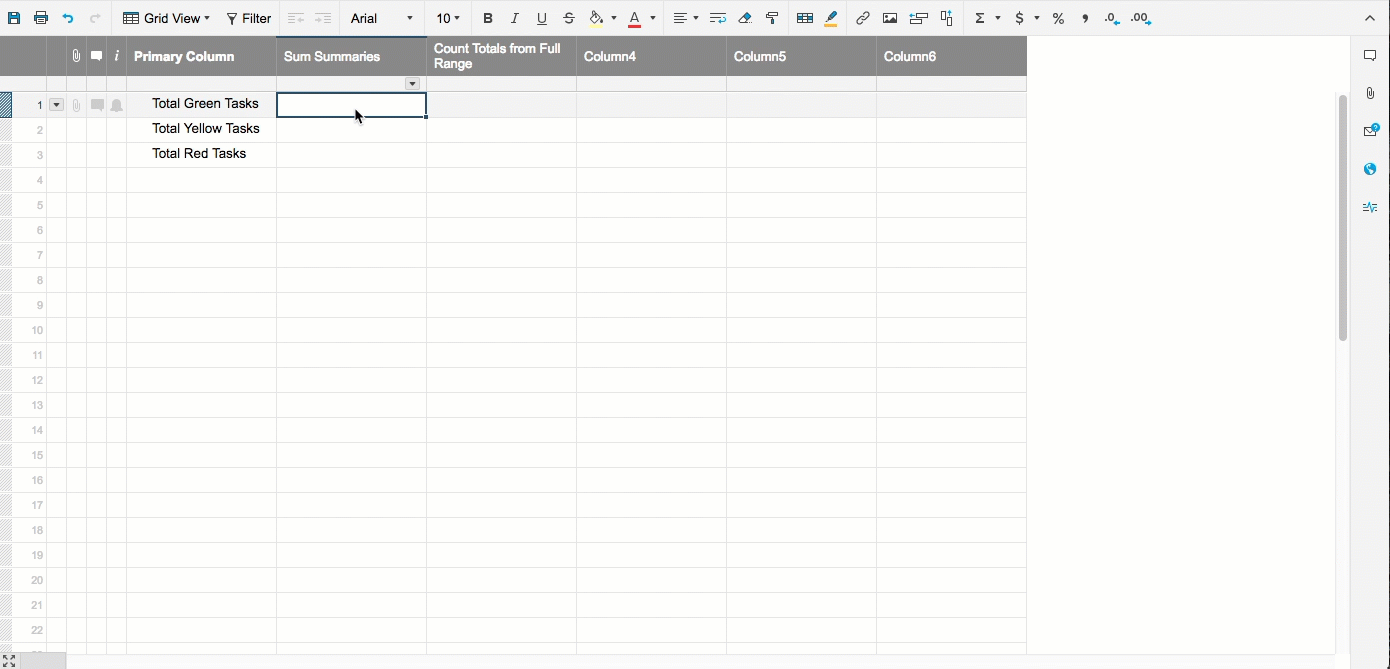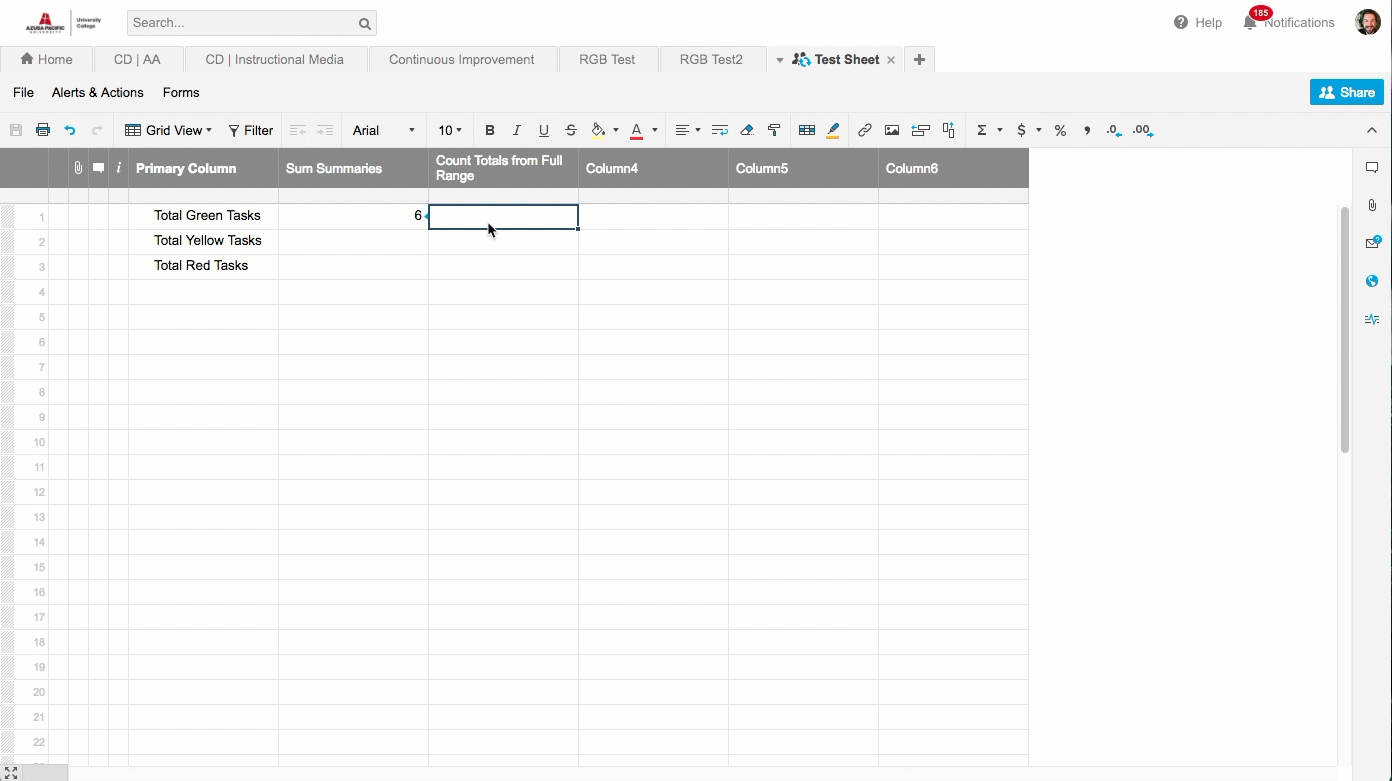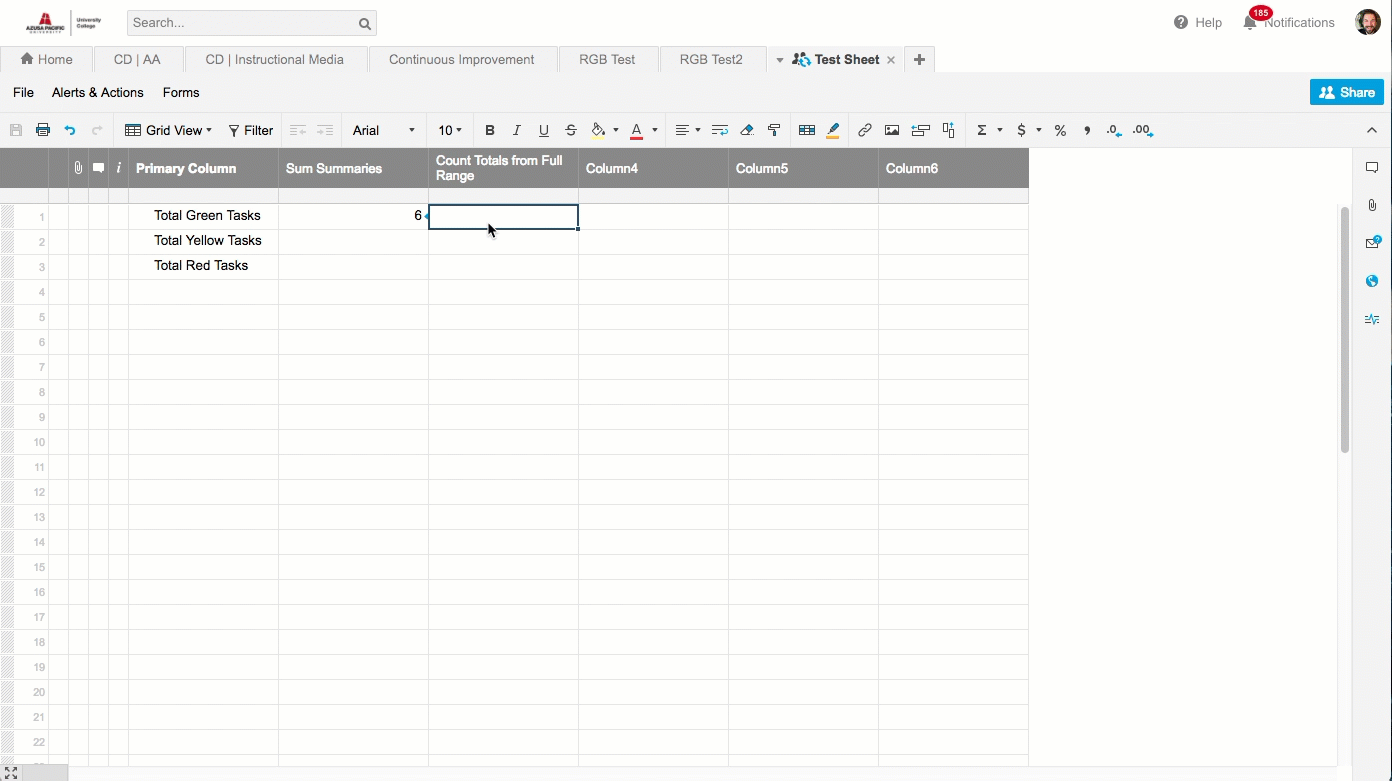# Count Task Status across Sheets

For each of our sheets, we use a "Health Status" of Red, Yellow, Green to indicate how that task is going. I would like to create a report which looks across all sheets and simply counts up the total number of tasks which are Red or Yellow. I can easily create the report which shows the list of individual tasks which are Red or Yellow but I cannot figure out how to "sum" this up.

My ultimate goal is to create a Dashboard Chart which will simply show this summed up number in a pie or bar chart but I need to get the report working first before I can make a chart out of it.

Thoughts?

Tags:

• Rather than count your data in a report, create a new spreadsheet and use an external reference to count the reds across each sheet...

=Countif({external range1}, "Red") + Countif({external Range 2}, "Red")

You just have to add the countifs together. Or if you have a summary cell already... then reference the summary cell either by linking it or creating it only as the range... then just use the =Sum() formula and reference each of those cells from another sheet. See my screenshots for more on that.• You can count the RGB across sheets in one of two ways.

1. Use cross sheet references to count the summary columns using the =Sum({cross refernce range}, {cross reference range2}, etc.)
2. Or by adding countifs that reference the RGB column together

=Countifs({Cross Reference Column Range1},"Green")+countifs({Cross Reference Range 2}, "Green")

Both will get you the same result you are looking for. See my screenshots for examples.## Help Article Resources

Want to practice working with formulas directly in Smartsheet?

Check out the Formula Handbook template!